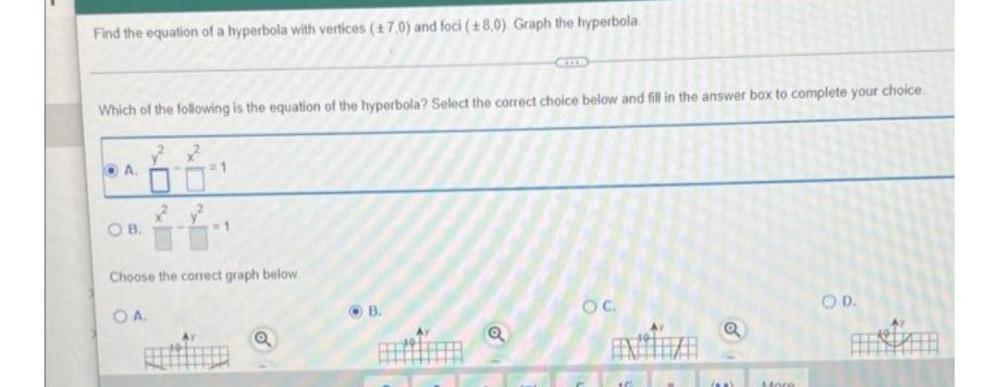Question:

# Find the equation of a hyperbola with vertices (170) and foci (8,0) Graph the hyperbola Which of the following is the equation oFind the equation of a hyperbola with vertices (170) and foci (8,0) Graph the hyperbola Which of the following is the equation of the hyperbola? Select the correct choice below and fill in the answer box to complete your choice OA 1 2 OB Choose the correct graph below. OC OD OA OB AY CERPEN